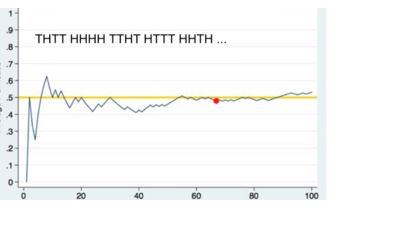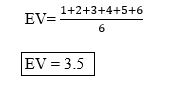### Law of Large Numbers

• date 26th June, 2019 |
• by Prwatech |

# Law of Large Numbers Tutorial

Law of large numbers tutorial, Are you the one who is looking for the best platform which provides information about the law of large numbers in data science, then welcome to the world of the law of large numbers statistics. In this tutorial, one can learn about the law of large numbers definition, why we need a law of large numbers in statistics and examples of the law of large numbers which are prepared by India’s largest Data science training institute in Bangalore professionals.

If you are the one who wanted to become an expert in Data Science? Or the one who wanted to explore the technology like a Pro under the certified experts with World-class classroom Training Environment, then Asks your Data Science Training institute experts who offer advanced Data Science training. Follow the below-mentioned law of large numbers tutorial and improve your skills to become professional Data Scientist.

## Law of Large Numbers Definition

It states that if you repeat an experiment independently a large number of times and average the result, what you obtain should be close to the expected value. i.e. In probability and statistics, states that as a sample size grows, its mean gets closer to the average of the whole population.

In the financial context, the law of large numbers indicates that a large entity that is growing rapidly cannot maintain that growth pace forever.The law of large numbers states, an observed sample average from a large sample will be close to the true population average and it will get closer to the larger sample.

The law of large numbers does not guarantee that a particular sample, especially a small sample, will reflect true population characteristics or that a sample which does not reflect the true population will be balanced by a subsequent sample.

In business, the term “law of large numbers” is sometimes used in a different sense to express the relationship between scale and growth rates.

### Why we need the Law of large numbers statistics?

The law of large numbers can be applied to a variety of subjects. It may not be feasible to poll every individual within a given population to collect the required amount of data, but every additional data point gathered has the potential to increase the likelihood that the outcome is a true measure of the mean.

If a person wanted to determine the average value of a data set of 100 possible values, he is more likely to reach an accurate average by choosing 20 data points instead of relying on just two. For example, if the data set included all integers from 1 to 100, and the sample taker only drew two values, such as 95 and 40, he may determine the average to be approximately 67.5. If he continued to take random samplings up to 20 variables, the average should shift towards the true average as he considers more data points.

## Example of Law of Large Numbers

The simplest example of the law of large numbers is rolling the dice. The dice involves six different events with equal probabilities. The expected value of the dice events is:If we roll the dice only three times, the average of the obtained results may be far from the expected value.

Let’s say you rolled the dice three times and the outcomes were 6, 6, 3.

The average of the results is 5.

According to the law of the large numbers, if we roll the dice a large number

of times, the average result will be closed the expected value of 3.5.

We hope you have understood the basics of the law of large numbers tutorial and its formula with examples in statistics. Interested in learning more? Then Get enroll with Prwatech for advanced Data science training in Bangalore with 100% placement assistance.

### Quick Support